﻿ Boolean Algebra for Xor-Gates

Boolean Algebra for Xor-Gates

Lianly RompisOPEN ACCESSPEER-REVIEWED

Boolean Algebra for Xor-Gates

Electrical Engineering Department, Universitas Katolik De la Salle, Manado, Indonesia

Abstract

Rules and laws of Boolean algebra are very essential for the simplification of a long and complex logic equation. Applying the Boolean algebra basic concept, such a kind of logic equation could be simplified in a more simple and efficient form.Mainly, the standard rules of Boolean algebra are given in operator ‘+’ and ‘x’, based on the AND and OR logic gates equations. For some logic designs, it is commonly that logic problems are writtenin terms of XOR format.This paper tries to conduct something different. It will analyze, describe, and derive Boolean algebra rules related to logic equations using exclusive-or (XOR) logic gate.

At a glance: Figures

1
Prev Next

• Rompis, Lianly. "Boolean Algebra for Xor-Gates." Journal of Computer Sciences and Applications 1.1 (2013): 14-16.
• Rompis, L. (2013). Boolean Algebra for Xor-Gates. Journal of Computer Sciences and Applications, 1(1), 14-16.
• Rompis, Lianly. "Boolean Algebra for Xor-Gates." Journal of Computer Sciences and Applications 1, no. 1 (2013): 14-16.

 Import into BibTeX Import into EndNote Import into RefMan Import into RefWorks

1. Introduction

In a major way, Boolean algebra differs from mathematics algebra. Boolean constants and variables are allowed to have only two logic values, 0 or 1, giving an output of these two possibilities [1, 2, 3].

Boolean Algebra is the algebra for digital signals. Standard rules and laws of this unique Algebra are derived from logic gates characteristics (AND-gate and OR-gate).Few characteristics are shown in Figure 1 and Figure 2.

Figure 1. Basic Multiplications for Boolean Algebra (characteristic of AND-gate)

From these basic rules, other rules are being derived as follows:(1)(2)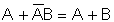(3)(4)

Beside rules, standard laws also have been set up for Boolean Algebra that generally use the same principles of mathematics algebra [2, 4, 5].

A. Commutative Law: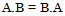(5)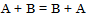(6)

B. Associative Law:(7)(8)

C. Distributive Law: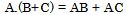(9)

Those rules and laws will offer you a great help when dealing with a complicated logic equations that need to be worked out.

2. Aims of Study

This paper is trying to derive rules of Boolean Algebra related to a logic equation of an exclusive-or (XOR) gate. There is a hope that this method will be useful for students, lecturers, and anyone that are interested in learning number system, or to whom that are taking subject related to computer or digital system.

3. Research Method

To derive the rules of Boolean Algebra related to an XOR gate, I take the basic point of standard rules and substitutes the standard rules with an XOR gate function

4. Discussion and Results

XOR-gate is a basic logic gate in digital system that use the combination of AND and OR gates.

The characteristic of XOR gate is given as follows: [1, 2, 3]

Table 1. XOR-Gate Truth TableDownload asPowerPoint Slide

Veiw figureView current table in a new window

If the number of logic 1s is Odd, the output will be 1, otherwise it will be 0.

Using the characteristic of XOR gate, substitutes the standard rules of Boolean Algebra with this function.If needed, apply Boolean Algebra laws.(10)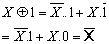(11)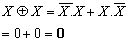(12)(13)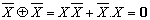(14)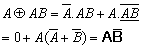(15)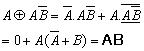(16)(17)(18)

So, we got the conclusion and rules for XOR Boolean algebra: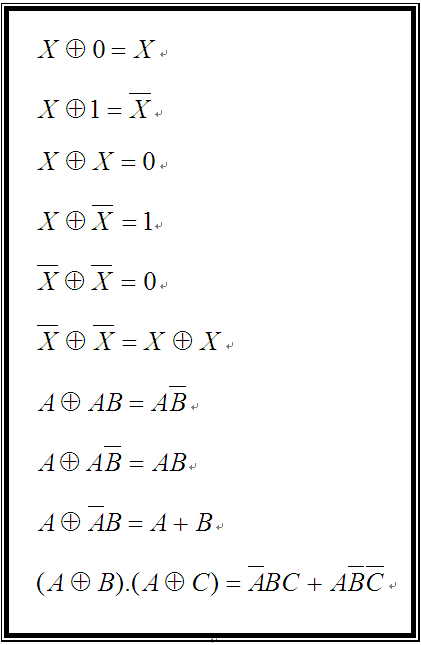5. Conclusion

Implementing XOR-gate characteristics into the standard rules and laws of Boolean algebra, will create a specific Boolean algebra that uses XOR function. It gives another way of solving logic equations which are written or given in XOR logic operator.

Acknowledgement

I would like to thank my colleagues and students for the moments and memories while working as a lecturer in my university.

References

  Malvino, A.P, ElektronikaKomputer Digital, PenerbitErlangga, Jakarta, 1988.In article  Mano, M.M., Computer System Architecture, 3rded.,PNJ: Prentice Hall, 1992.In article  Tokheim, R.L.,Prinsip-Prinsip Digital,PenerbitErlangga, Jakarta, 1996.In article  CIMT-Centre for Innovation in Mathematics Teaching, Boolean Algebra, University of Plymouth, 2005. Available: http://www.cimt.plymouth.ac.uk/projects/mepres/alevel/discrete_ch11.pdf.In article  Katzen, S.J., EEE111J2 Digital Systems: Boolean Algebra and Karnaugh Maps, University of Ulster, Ireland, 2007. Available: http://katzen.me.uk/Books/sidssite/eee111a/digital_4.pdf.In article